# Quantum Wells, Wires and Dots

## Quantum dot (pyramid)What you can learn:
• 3D simulation, quantum dot, strain, piezoelectric fields
• Strain equation, Poisson equation, Schrödinger equation in 3D

## Quantum dot molecule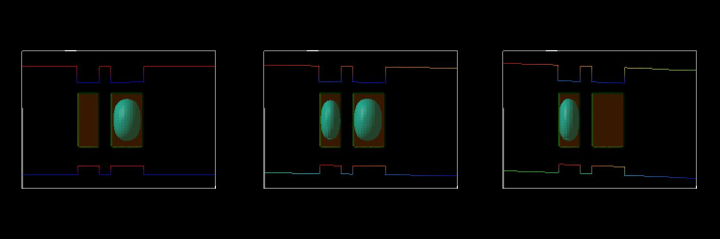What you can learn:
• 3D simulation, quantum dot, applying an electric field, performing an electric field sweep
• Schrödinger equation in 3D

## Quantum Dot Tutorials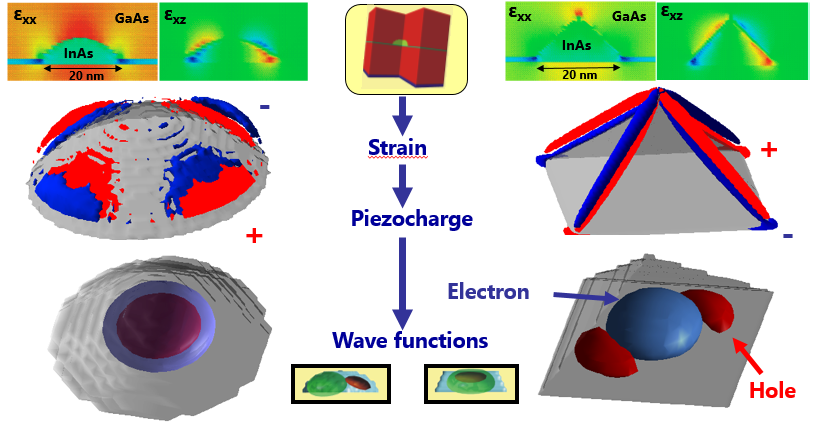## Coupled quantum wires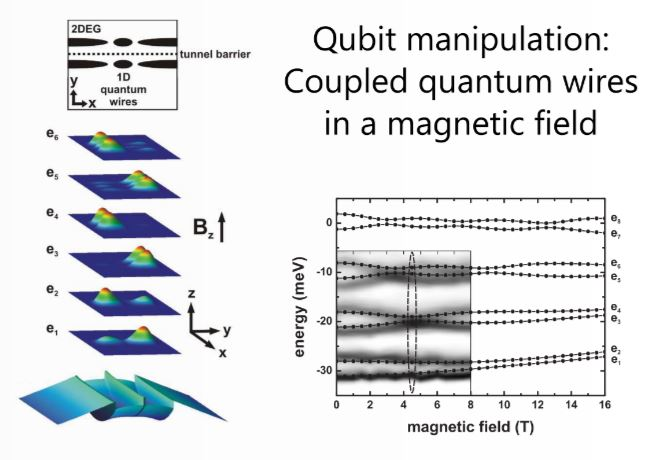Coupled quantum wires
One-dimensional electron transport in combination with wavefunction engineering has been proposed for the use in solid state quantum information processing. An example of such a system is studied in this tutorial:
Vertically coupled quantum wires in a longitudinal magnetic field

## AlGaInP on GaAs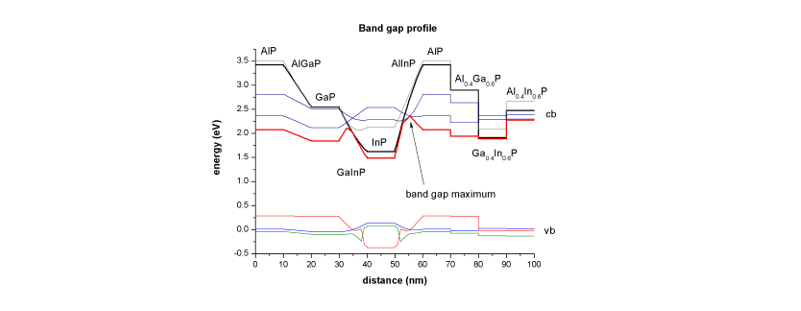What you can learn:
• alloys, linearly graded alloys, bowing, strain, band gaps
• strain equation

## Wurtzite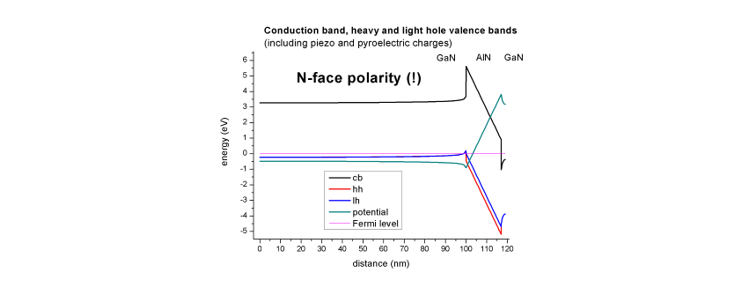What you can learn:
• wurtzite, strain, piezoelectricity, pyroelectricity
• strain equation, Poisson equation

## Optical absorption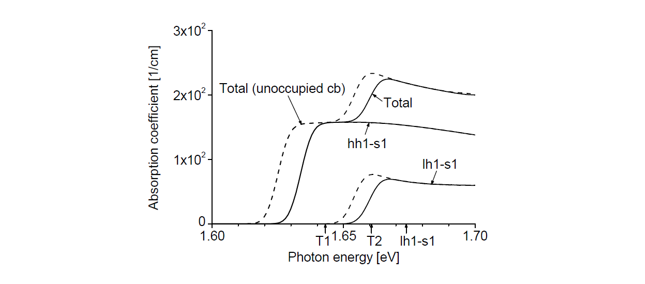Optical absorption of a quantum well - Diploma thesis documentation available

What you can learn:
• 8-band k·p, optical absorption
• 8-band k·p equation

## Core-shell nanowires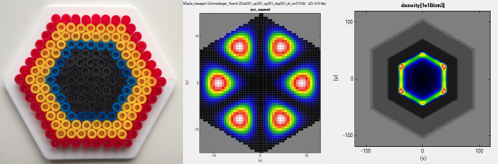What you can learn:
• 2D simulations, circular and hexagonal quantum confinement, core-shell nanowires, sweep over alloy concentrations and core radius, interband transitions (spatial overlap matrix elements)
• Schrödinger equation in 2D, self-consistent Schrödinger-Poisson equation in 2D

## Broken gap quantum well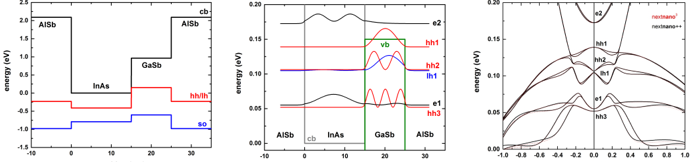InAs / GaSb broken gap quantum well (BGQW) (type-II band alignment)

What you can learn:
• 1D simulation, 8-band k·p, broken gap, type-II band alignment
• 8-band k·p equation